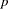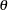The BTL Procedure

MARKER variables </ options> ;

The MARKER statement lists the genetics marker variables used in constructing the BTL model. This statement is required, and at least one marker variable must be listed or an error will be generated. The MARKER statement is similar to a class statement, except the interaction effect for all the marker variables listed is implicitly added to the model, as in the following code:

```   proc btl;
marker m1 m2 m3;
model trait;
run;
```

The model trait = m1m2m3 is fit by PROC BTL. Additionally, if the PARMEST statement is used, these markers are included in the calculated BTL model and the model recombination and penetrance parameters are estimated.

You can specify the following options in the MARKER statement after a slash (/).

ALL=number

requests that regression models be calculated for all combinations of number markers from the marker statement, where number is a positive integer. Note:If the ALL option is used, the PARMEST statement is ignored if specified. If this option and the ALLUPTO= option are omitted, then only a model including all markers is fit. This option cannot be used if ALLUPTO= is specified.

ALLUPTO=number

requests that regression models be calculated for all combinations of number and fewer markers from the marker statement; e.g., ALLUPTO=3 calculates all combinations of 3, 2, and 1 markers, where number is a positive integer. Note:If the ALLUPTO option is used, the PARMEST statement is ignored if specified. If this option and the ALL= option are omitted, then only a model including all markers is fit. This option cannot be used if ALL= is specified.

BEST=number

requests that only the top number models be included in the output, where number is a positive integer. By default, all models are displayed. If the GROUP= option is used, number specifies the number of models per linkage group to include in the output. The ranking of the models is determined using the model selection criterion selected using the MC= option or the-value of the likelihood ratio test by default.

GROUP=variable

specifies the name of the linkage group variable in the map data set to be used in grouping the models in the "Model Statistics" output if the output contains single-gene models only. The output contains the models sorted by linkage group and by the criterion specified in the MC= option (or the likelihood ratio-value by default). If thes are not specified using the THETA option but are calculated using the MAP= data set, thens between markers from different linkage groups are set to 0.5. Note:If this variable is specified, markers in the MAP= data set from the same linkage group must be in consecutive observations. Values for this variable must be nonnegative numbers; any negative values are treated as missing.

MC=P
MC=LRCHI
MC=AIC
MC=BIC
MC=AICC

specifies the model selection criterion used to sort models. The default value is the-value from the likelihood ratio test (MC=P).

Note: This procedure is experimental.Previous Page | Next Page | Top of Page